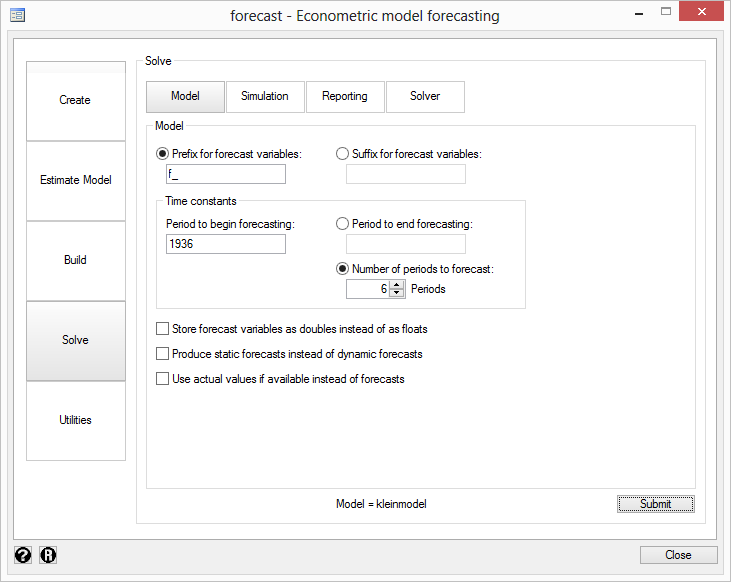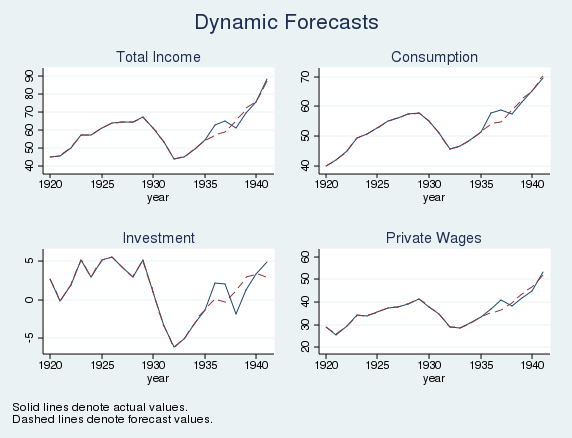Home  /  Products  /  Stata 13  /  Forecasting

## Forecasting

### Highlights

 Time-series and panel datasets Multiple estimation results OLS, VARs, VECs, ARIMAs, ARCHs, 3SLS, and more Estimated with Stata or obtained from outside sources One equation or thousands Identities Add factors and other adjustments Dynamic or static (one-step-ahead) forecasts Solve simultaneous systems Confidence intervals via stochastic simulation Compare forecasts of alternative scenarios

### Show me

We will forecast a simple seven-equation model produced by one estimation command, but we could forecast a model with thousands of equations obtained from thousands of estimation commands.

We fit a classic model (Klein 1950) of the US economy to pre-World War II data using 3SLS:

. reg3 (c p L.p w) (i p L.p L.k) (wp y L.y yr), endog(w p y) exog(t wg g)
(output omitted)

. estimates store klein


Now we tell forecast about our estimation results and the model's identities:

. forecast create kleinmodel Forecast model kleinmodel started. . forecast estimates klein Added estimation results from reg3. Forecast model kleinmodel now contains 3 endogenous variables. . forecast identity y = c + i + g Forecast model kleinmodel now contains 4 endogenous variables. . forecast identity p = y - t - wp Forecast model kleinmodel now contains 5 endogenous variables. . forecast identity k = L.k + i Forecast model kleinmodel now contains 6 endogenous variables. . forecast identity w = wg + wp Forecast model kleinmodel now contains 7 endogenous variables. . forecast exogenous wg g t yr Forecast model kleinmodel now contains 4 declared exogenous variables.

Even better, we could have entered the above into forecast's Control Panel:The result is

. forecast solve, begin(1936) Forecast 7 variables spanning 6 periods. Starting period: 1936 Ending period: 1941 Forecast prefix: f_ 1936: ............................................ 1937: .......................................... 1938: ............................................. 1939: ............................................. 1940: ............................................ 1941: ..............................................

That may look boring, but forecast has saved our forecast. We could graph the results:Notice how easy this all was. We used reg3 to fit stochastic equations for three variables, and we stored our estimation results. We then created a forecast model named kleinmodel and added those results. This model has only one set of estimation results, but we could have added more. We then defined a few identities that describe other variables in our model. The first one is familiar to introductory economics students: the nation's income is the sum of consumption, investment, and government spending (ignoring foreign trade). This period's capital stock is just last year's capital stock plus net investment, where investment was one of the equations in our 3SLS model. We told forecast about our exogenous variables; then we told it to solve our model.

By default, forecast produces dynamic forecasts, but we could have asked for static ones instead. Moreover, we could have asked forecast to estimate the amount of error surrounding our forecast by accounting for variability stemming from parameter uncertainty as well as additive error terms.

forecast also lets you specify alternative paths for some variables and obtain forecasts for the other variables in the model conditional on those paths. That way, you can perform policy simulations and develop forecasts assuming a range of different scenarios.

### Show me more

Learn much more about forecasting in Stata by reading the manual entry.

See New in Stata 18 to learn about what was added in Stata 18.